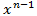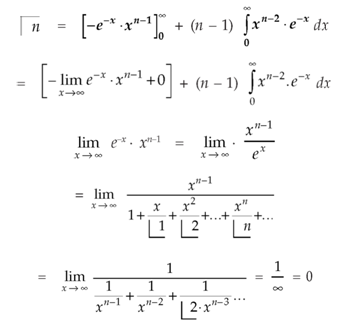Goseeko blog# What are beta and gamma functions?

## Overview

As we know, functions play an important role in mathematics. A function defines a specific correlation between the two data sets. Beta and gamma functions are from the group of Euler’s integral functions.

In this blog we will discuss the beta and gamma function, their properties, relationship between the two and problems based on these functions.

The study of beta and gamma functions is very useful in the areas of mathematics and science.

We use this concept in string theory, complex physics, integral calculus,  etc.

## Beta functions

This is the first kind of Euler’s integral, this function has real number domain,.

The beta function is-

## Gamma function

The gamma function is an improper integral, which is dependent on n,

This is defined as the second kind of Euler’s integral

Note- Beta function is symmetrical with respect to m and n.

Some important results-

The result is known as the recurrence formula for gamma function.

Note-

1.     Symmetry-

2.     Beta function in terms of trigonometric functions can be written as-

Evaluation of beta function ? (m, n)

Here we have-

Or

Again integrate by parts, we get-

Repeating the process above, integrating by parts we get-

or

Evaluation of gamma function

Integrating by parts, we takeas first function-We get

Replace n by n+1,

Example: Evaluate

Sol:

Example: Find the value of

Sol:

We know that

So that

Interested in learning about similar topics? Here are a few hand-picked blogs for you!